# Potential

(diff) ← Older revision | Latest revision (diff) | Newer revision → (diff)

potential function

A characteristic of a vector field.

A scalar potential is a scalar function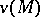such that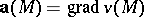at every point of the domain of definition of the field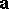(sometimes, for example in physics, its negative is called a potential). If such a function exists, the vector field is called a potential field.

A vector potential is a vector function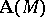such that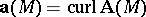(cf. Curl) at every point of the domain of definition of the field. If such a function exists, the vector fieldis called a solenoidal field.

Depending on the distribution of the mass or the charge by which the potential is generated one speaks about a potential of a point-charge, a surface potential (single-layer or double-layer), a volume potential, etc. (see Potential theory).

The use of a vector potential is restricted to three-dimensional vector fields. In this case one can prove the so-called Clebsch lemma, according to which any vector field can be represented as a sum of a potential field and a solenoidal field,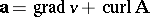.